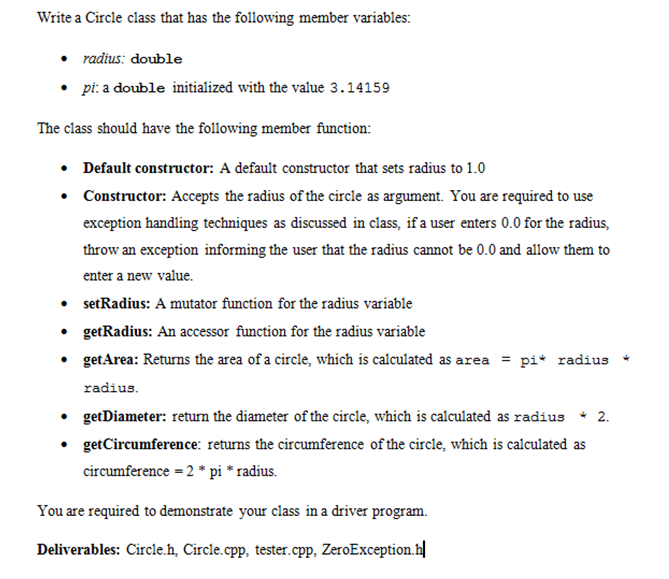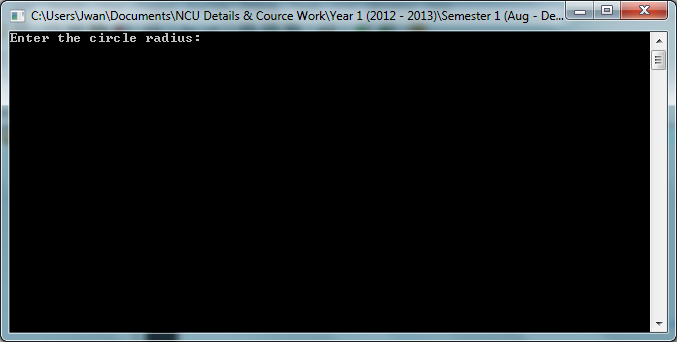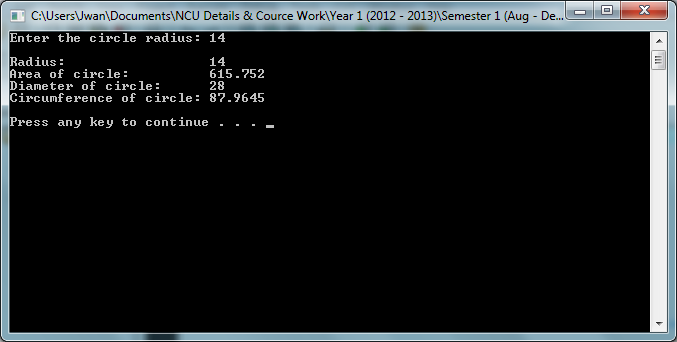Circle (using classes and exception handler)Posted by JanWan Last Updated: November 13, 20122714J.W. PRODUCTIONI'm currently working on this question...Check this out. http://sourcecodeera.com/blogs/Samath/Circle-Class-in-C.aspxThe interface for this question (Circle.h) class Circle#include #include using namespace std; class Circle {       private: //Private member               double radius; //Declare variable                      public: //Public data members              void setRadius(double); //Mutator              double getRadius(); //Accessor                            //Other member function              double getArea();              double getDiameter();              double getCircumference();                           //Constructors              Circle(double);              Circle(); };This is the implementation of the circle Circle.cpp#include #include #include #include "Circle.h" #include #include "ZeroException.h" using namespace std; const double pi = 3.14159; //Radius function/ Mutator void Circle::setRadius(double R) {      //Throw exception if radius is 0      if(R == 0.0)      {                throw ZeroException(); //Throw an exception      }            radius = R; //set R value to variable radius } //Radius function/ Accessor double Circle::getRadius() {      return radius; //return value of radius } //Area function double Circle::getArea() {        double area; //Declare variable        return area = pi * radius * radius; //calculation of area } //Diameter function double Circle::getDiameter() {        double dia; //Declare variable        return dia = radius * 2; //calculate and return diameter } //Circumference function double Circle::getCircumference() {        double CirF; //Declare variable        return CirF = 2 * pi * radius; //Calculate and return the circumference } //Constructor with argument Circle::Circle(double R) {      setRadius(R); //set variable in constructor } //Default constructor Circle::Circle() {       radius = 1.0; //set radius to 1.0 } J.W. PRODUCTIONMain/Tester for the program#include #include #include #include "Circle.h" #include "ZeroException.h" using namespace std; int main(int argc, char *argv[]) {     double rad; //Declare variable          cout<<"Enter the circle radius: ";          //Try contain code that might cause exception and code that     //should not execute if exception occur     try     {        cin>>rad; //input value        Circle cork(rad); //instant of the class        cout<Screen Shot for Class Circle Program Enter the value radiusResults of RadiusError message from Exception Handling Zero can not be enter as a valid value